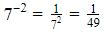Courses

# Test: Exponents And Powers- 3

## 20 Questions MCQ Test Mathematics (Maths) Class 8 | Test: Exponents And Powers- 3

Description
This mock test of Test: Exponents And Powers- 3 for Class 8 helps you for every Class 8 entrance exam. This contains 20 Multiple Choice Questions for Class 8 Test: Exponents And Powers- 3 (mcq) to study with solutions a complete question bank. The solved questions answers in this Test: Exponents And Powers- 3 quiz give you a good mix of easy questions and tough questions. Class 8 students definitely take this Test: Exponents And Powers- 3 exercise for a better result in the exam. You can find other Test: Exponents And Powers- 3 extra questions, long questions & short questions for Class 8 on EduRev as well by searching above.
QUESTION: 1

### What is the value of (30−40) × 5−3 ?

Solution:

Since ao = 1 for any 'a', (30−40) × 5−3 = (1−1) × 5−3 = 0

QUESTION: 2

### Expand 1256.249 using exponents.

Solution:

⇒ (1 x 1000) +(2 x 100) + (5 x 10) + (6 x 1) + (2/10) + (4/100) + (9/1000) .

⇒ (1 x 103) + (2 x 102) + (5 x 101) + (6 x 100) + (2 x 10-1) + (4 x 10-2) +(9 x 10-3) (Ans)

QUESTION: 3

### What is the solution of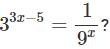Solution: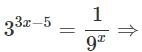33x−5 = 3−2x ⇒ 3x−5 =−2x

[When bases are equal, powers can be equated.]

⇒ 5x = 5

⇒ x = 1

QUESTION: 4

Simplify: (−3)2×(5/3)2

Solution:

(-3)x (5/3)= (-1)2(3)2-2(25) = 25

QUESTION: 5

Write the expression using exponents: 89 × 89 × 89 × 89

Solution:
QUESTION: 6

Evaluate exponential expression − 25.

Solution:
QUESTION: 7

The value of 23 is _____.

Solution:

23 = 2x2x2 = 8

QUESTION: 8

Simplify and write in exponential form: p3×p−10

Solution:
QUESTION: 9

Very small numbers can be expressed in standard form using __________ exponents.

Solution:
QUESTION: 10

a= _______

Solution:
QUESTION: 11

The area of a square is given by the formula A = c2. What will be the total area of 5 such similar squares, if the side of a square is 8 ft?

Solution: We have,length of each side of a square, s = 8 ftarea of 1 square = s^2 = 8 x 8 = 64 ft^2Now, area of 5 squares = 5 x 64 = 320 ft^2
QUESTION: 12

Evaluate the exponential expression (−n)4× (−n)2, for n = 5.

Solution: (-n)^4×(-n)^2for n =(-5)^4×(-5)^2 by formula if(a)^n×(a)^m=a^m+n =(-5)^4+2 =-5^6 =5×5×5×5×5×5 =15625
QUESTION: 13

The area of a square is given by the formula A = side2. What will be the total area of 5such similar squares, if the side of a square is 7 in?

Solution:

Side of the square is 7 inches
So area of one square is  7= 49
Total area of 5 such squares is
49×549×5 = 245 in2

QUESTION: 14

Which of the following is the value of (4 / 5)-9 / (4 / 5)-9?

Solution: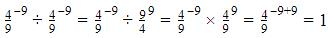QUESTION: 15

Simplify: 25÷ 2−6

Solution:

25/2-6 = 25-(-6) = 211

QUESTION: 16

Simplify: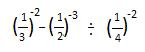Solution:
QUESTION: 17

Find the value of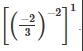Solution:
QUESTION: 18

Find the value of the expression a2 for a = 10.

Solution:

a = 10, a2 = 102
10x10 =100

QUESTION: 19

Evaluate: - 73

Solution:
QUESTION: 20

Find the multiplicative inverse of 7−2.

Solution: